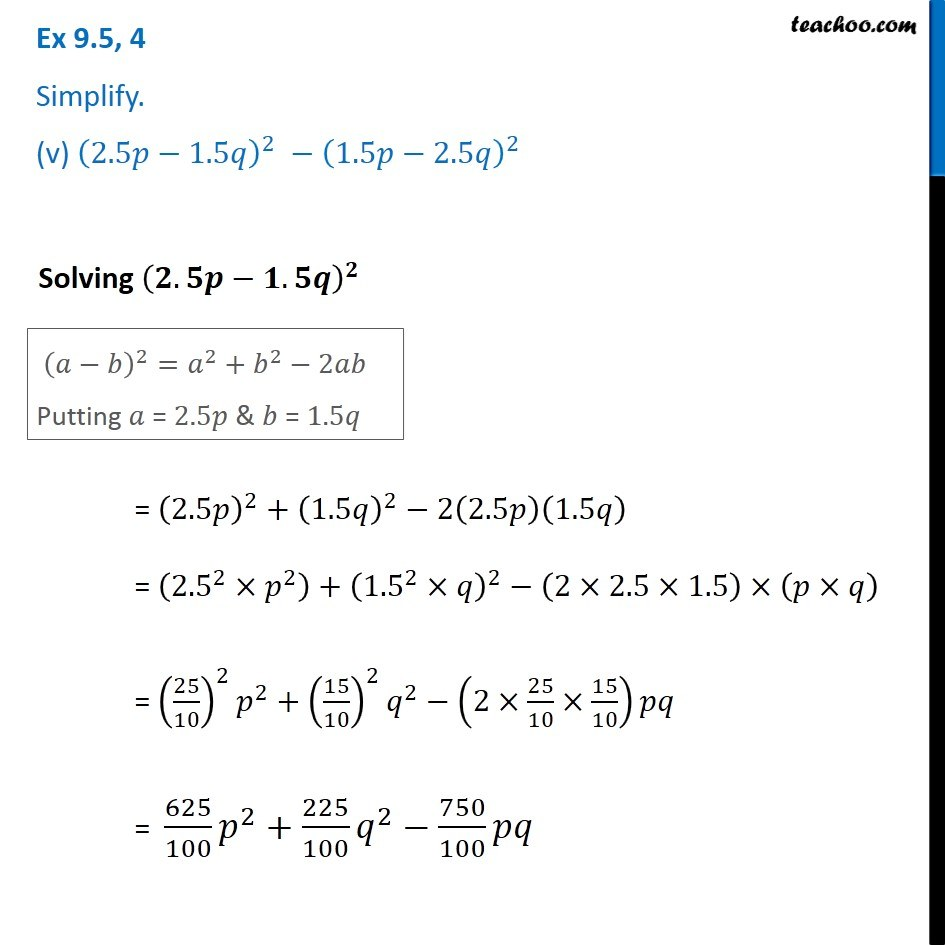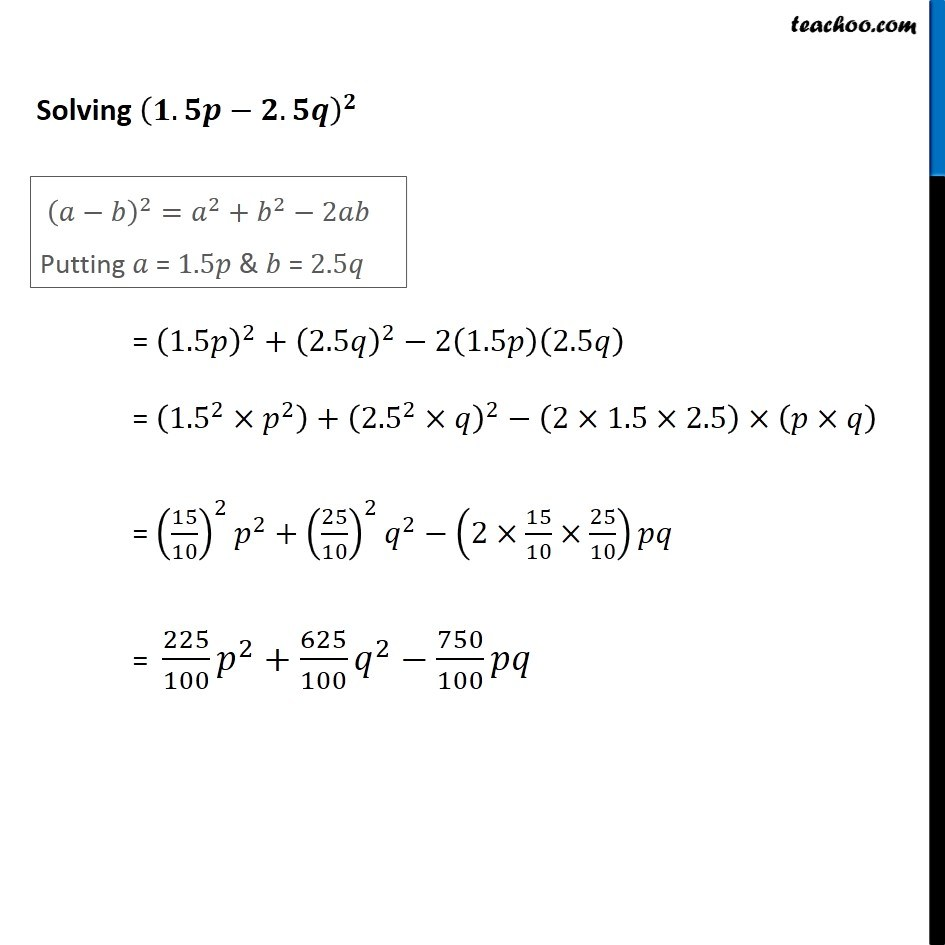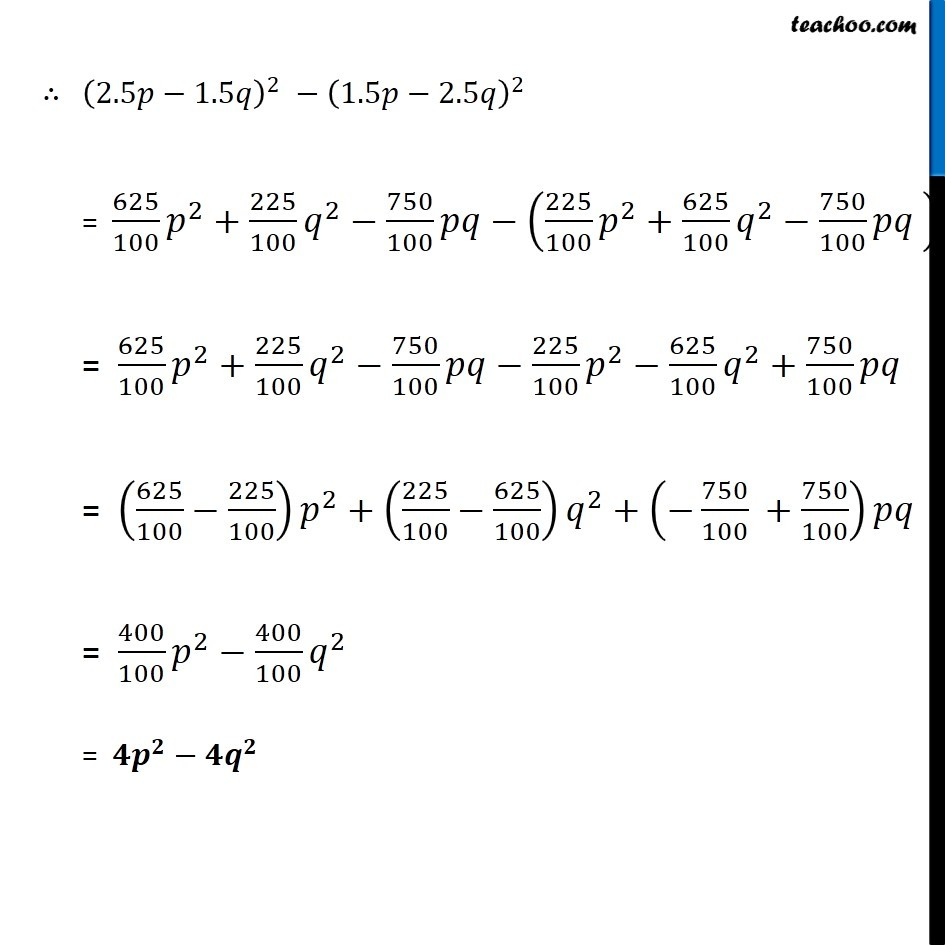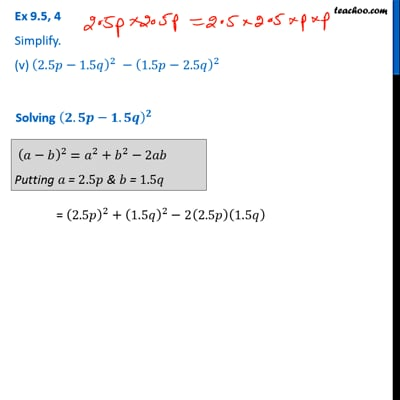Algebra Identities and Formulas

Chapter 8 Class 8 Algebraic Expressions and Identities
Serial order wiseThis video is only available for Teachoo black users

Learn in your speed, with individual attention - Teachoo Maths 1-on-1 Class

### Transcript

Question 4 Simplify. (v) (2.5𝑝−1.5𝑞)^2 −(1.5𝑝−2.5𝑞)^2 Solving (𝟐.𝟓𝒑−𝟏.𝟓𝒒)^𝟐 (𝑎−𝑏)^2=𝑎^2+𝑏^2−2𝑎𝑏 Putting 𝑎 = 2.5𝑝 & 𝑏 = 1.5𝑞 = (2.5𝑝)^2+(1.5𝑞)^2− 2(2.5𝑝)(1.5𝑞) = (〖2.5〗^2×𝑝^2 )+(〖1.5〗^2×𝑞)^2 − (2×2.5×1.5)×(𝑝×𝑞) = (25/10)^2 𝑝^2+(15/10)^2 𝑞^2 − (2×25/10×15/10)𝑝𝑞 = 625/100 𝑝^2+225/100 𝑞^2−750/100 𝑝𝑞 Solving (𝟏.𝟓𝒑−𝟐.𝟓𝒒)^𝟐 (𝑎−𝑏)^2=𝑎^2+𝑏^2−2𝑎𝑏 Putting 𝑎 = 1.5𝑝 & 𝑏 = 2.5𝑞 = (1.5𝑝)^2+(2.5𝑞)^2−2(1.5𝑝)(2.5𝑞) = (〖1.5〗^2×𝑝^2 )+(〖2.5〗^2×𝑞)^2−(2×1.5×2.5)×(𝑝×𝑞) = (15/10)^2 𝑝^2+(25/10)^2 𝑞^2−(2×15/10×25/10)𝑝𝑞 = 225/100 𝑝^2+625/100 𝑞^2−750/100 𝑝𝑞 ∴ (2.5𝑝−1.5𝑞)^2 −(1.5𝑝−2.5𝑞)^2 = 625/100 𝑝^2+225/100 𝑞^2−750/100 𝑝𝑞−(225/100 𝑝^2+625/100 𝑞^2−750/100 𝑝𝑞" " ) = 625/100 𝑝^2+225/100 𝑞^2−750/100 𝑝𝑞−225/100 𝑝^2−625/100 𝑞^2+750/100 𝑝𝑞 = (625/100−225/100) 𝑝^2+(225/100−625/100) 𝑞^2+(−750/100 +750/100)𝑝𝑞 = 400/100 𝑝^2−400/100 𝑞^2 = 𝟒𝒑^𝟐−𝟒𝒒^𝟐